# Problem 51783. Solve an ODE: equation B

Write a function to solve the following ordinary differential equation:with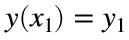and either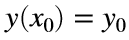or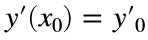. Along with y1, one of y0 and yp0 will be assigned numerical values, and the other will be NaN. The function should return the values of y at the specified values of x.
One of the applications for this equation is in groundwater. For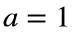and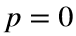the equation arises in the flow of water to a well pumping in a leaky confined aquifer; the independent variable x is the normalized distance from the well, and y is related to the piezometric head, a combination of the elevation and pressure of the water. Specifying the derivative at a point amounts to specifying the flow to the well.

### Solution Stats

42.42% Correct | 57.58% Incorrect
Last Solution submitted on Jul 18, 2023

### Community Treasure Hunt

Find the treasures in MATLAB Central and discover how the community can help you!

Start Hunting!